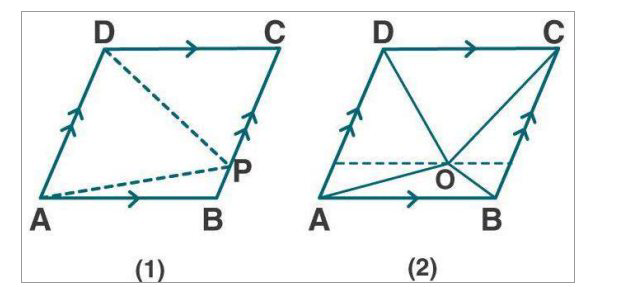# ML Aggarwal Solutions Class 9 Mathematics Solutions for Theorems on Area Exercise 14 in Chapter 14 - Theorems on Area

(a) In the figure (1) given below, ABCD is a parallelogram and P is any point in

BC. Prove that: Area of ∆ABP + area of ∆DPC = Area of ∆APD.

(b) In the figure (2) given below, O is any point inside a parallelogram ABCD. Prove

that:

(i) area of ∆OAB + area of ∆OCD = ½ area of || gm ABCD

(ii) area of ∆ OBC + area of ∆ OAD = ½ area of || gm ABCD(a) Given:

From fig (1)

ABCD is a parallelogram and P is any point in BC.

To prove:

Area of ∆ABP + area of ∆DPC = Area of ∆APD

Proof:

∆APD and || gm ABCD are on the same base AD and between the same || lines AD and

BC,

ar (∆APD) = ½ ar (|| gm ABCD) …. (1)

In parallelogram ABCD

ar(|| gm ABCD) = ar (∆ ABP) + ar (∆APD) + ar (∆DPC)

Now, divide both sides by 2, we get

½ ar(|| gm ABCD) = ½ ar (∆ ABP) + ½ ar (∆APD) + ½ ar (∆DPC) …. (2)

From (1) and (2)

ar (∆APD) = ½ ar (|| gm ABCD)

Substituting (2) in (1)

ar (∆APD) = ½ ar (∆ ABP) + ½ ar (∆APD) + ½ ar (∆DPC)

ar (∆APD) - ½ ar (∆APD) = ½ ar (∆ ABP) + ½ ar (∆DPC)

½ ar (∆APD) = ½ [ar (∆ ABP) + ar (∆DPC)]

ar (∆APD) = ar (∆ ABP) + ar (∆DPC)

Or ar (∆ ABP) + ar (∆DPC) = ar (∆APD)

Hence proved.

(b) Given:

From fig (2)

|| gm ABCD in which O is any point inside it.

To prove:

(i) area of ∆OAB + area of ∆OCD = ½ area of || gm ABCD

(ii) area of ∆ OBC + area of ∆ OAD = ½ area of || gm ABCD

Draw POQ || AB through O. It meets AD at P and BC at Q.

Proof:

(i) AB || PQ and AP || BQ

ABQP is a || gm

Similarly, PQCD is a || gm

Now, ∆OAB and || gm ABQP are on same base AB and between same || lines AB and PQ

ar (∆OAB) = ½ ar (||gm ABQP) …. (1)

Similarly, ar (∆OCD) = ½ ar (||gm PQCD) …. (2)

Now by adding (1) and (2)

ar (∆OAB) + ar (∆OCD) = ½ ar (|| gm ABQP) + ½ ar (|| gm PQCD)

= ½ [ar (|| gm ABQP) + ar (|| gm PQCD)]

= ½ ar (|| gm ABCD)

ar (∆OAB) + ar (∆OCD) = ½ ar (|| gm ABCD)

Hence proved.

(ii) we know that,

ar (∆OAB) + ar (∆ OBC) + ar (∆OCD) + ar (∆OAD) = ar (|| gm ABCD)

[ar (∆OAB) + ar (∆OCD)] + [ar (∆ OBC) + ar (∆OAD)] = ar (|| gm ABCD)

½ ar (|| gm ABCD) + ar (∆OBC) + ar (∆OAD) = ar (|| gm ABCD)

ar (∆OBC) + ar (∆OAD) = ar (|| gm ABCD) - ½ ar (|| gm ABCD)

ar (∆OBC) + ar (∆OAD) = ½ ar (|| gm ABCD)

Hence proved.

Related Questions
Exercises

Lido

Courses

Teachers

Book a Demo with us

Syllabus

Maths
CBSE
Maths
ICSE
Science
CBSE

Science
ICSE
English
CBSE
English
ICSE
Coding

Terms & Policies

Selina Question Bank

Maths
Physics
Biology

Allied Question Bank

Chemistry
Connect with us on social media!